## Thursday, May 2, 2019

 table div table+table+table div table{width:100%;padding:0}table div table+table+table div table img{width:96.23%;padding:0;float:none}table div table+table+table div table td{width:100%;padding:0 1.88% 18px}/* styles */# Sunday's Service - May 5

 /* styles */ We welcome back Reverend Bruce Irwin to lead us in Worship this week. The featured Scripture verses are: John 21:1-19 for Bruce's message: Let's Not Miss God's Next Call in Our Lives.
 /* styles */ ~~~

# CG NEWS & EVENTS## Paperless Service

We are a little late for Earth Day, but we will practice creation care this Sunday by having a "green" service and taking a bulletin break. The order of Worship will proceed as normal. Following are the songs for this week:
Songs of Worship - You're Worthy of My Praise and You Are My All in All
Closing Song - Thy Word## Bible Studies

Sunday Morning Class: Romans (No class this week.)
Wednesday Morning Ladies Study: Hosea, 9:30am at Jen Shannon's

You are welcome to join in studies at any time!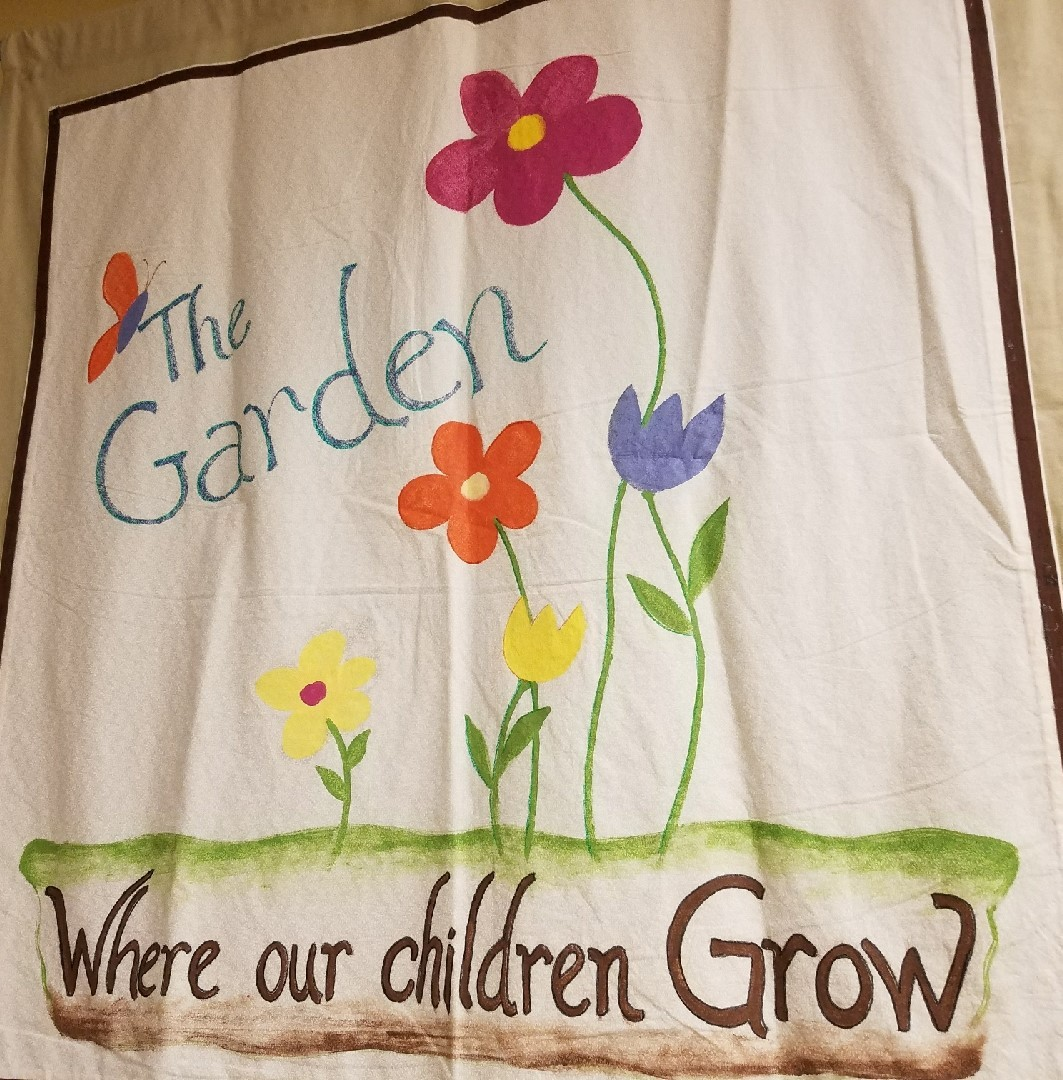## Garden & Nursery News

Jennifer Lynch will be in the Garden this week, with a lesson about worries and hope. Activity pages will be on the table by the Sanctuary doors. Children 3 & younger are invited to the Nursery for Bible story time.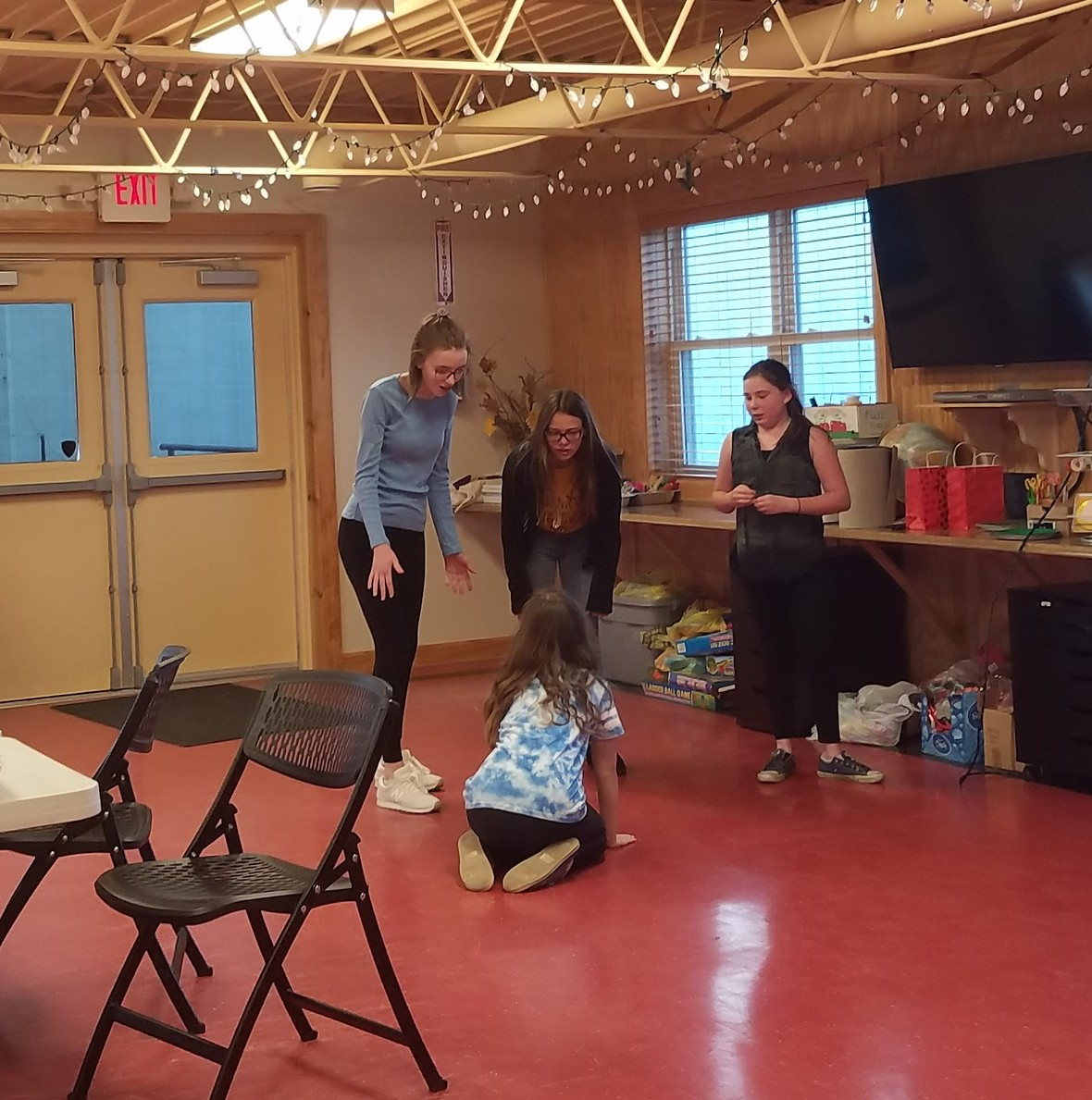## Youth Group

Last Sunday we talked about faith and the healing of the blind man and spent time praying for other's worries. We also enjoyed a charades race. Games and discussions with these kids are always interesting!
Hope to see all our youth this Sunday from 6-7:30pm! We will not meet on Mother's Day. Our final school year meeting will be on May 19th. Summer plans to come soon.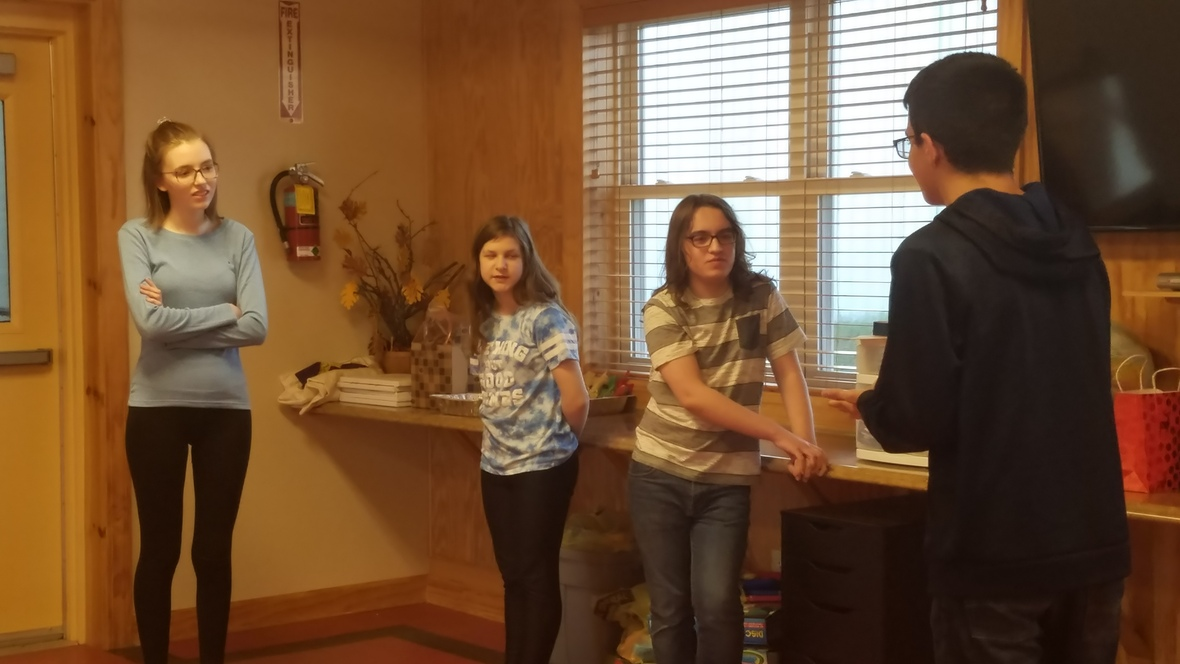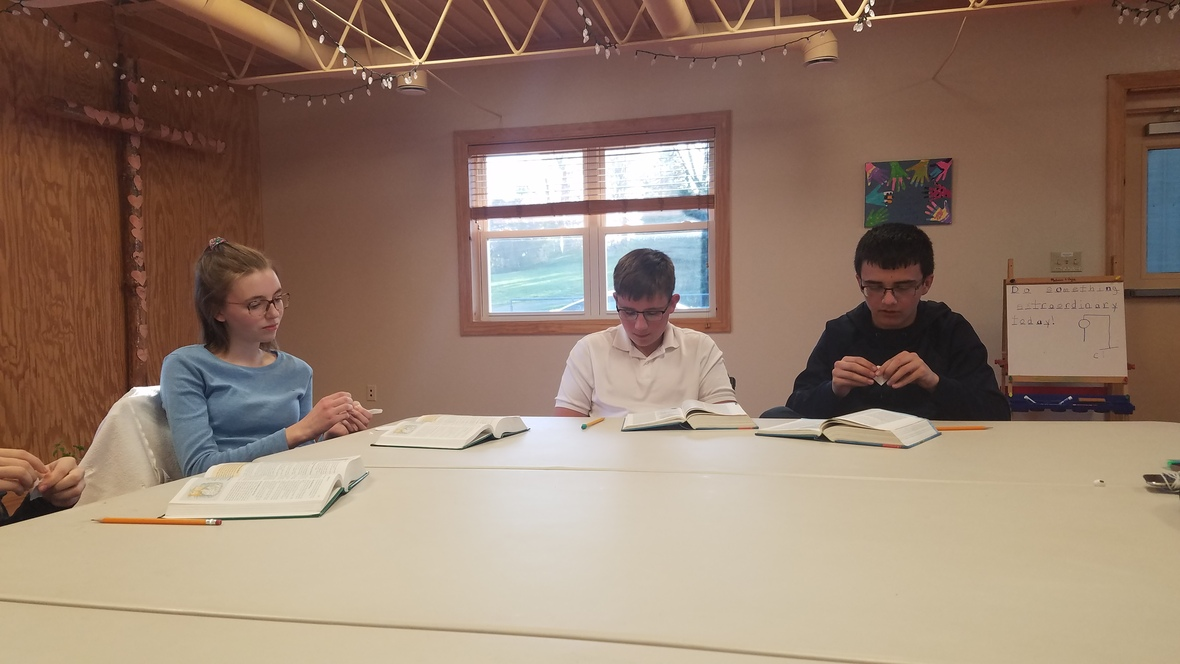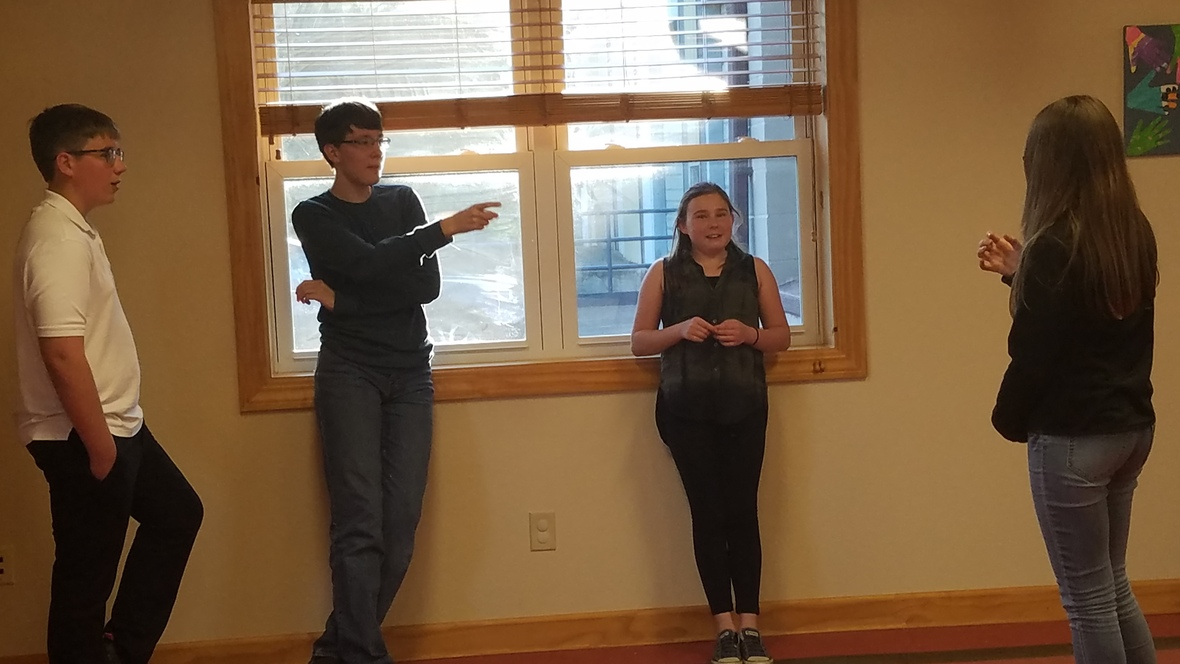table div table+table+table+table+table+table+table+table+table+table+table+table+table+table div table{width:100%;padding:0}table div table+table+table+table+table+table+table+table+table+table+table+table+table+table div table img{width:96.23%;padding:0;float:none}table div table+table+table+table+table+table+table+table+table+table+table+table+table+table div table td{width:100%;padding:0 1.88% 18px}/* styles */# UPCOMING AT CG## May & June Speakers

May 12 - Pat Shannon
May 26 - Adam Shank - Communion Sunday
June 2 - Phil Bartholomew
June 9 - Phil Bartholomew
June 16 - John Muckridge

## Welcome Summer Potluck

Celebrate summer after Worship on June 23 with hot dogs and an ice cream sundae bar. Bring your favorite summer food to share. Sign up sheet to come in the beginning of June.

August 24 ~ Canning Class: Spaghetti Sauce, Time TBD
October 12 ~ Outreach Coat & Clothing Giveaway, 10am-1pm

 table div table+table+table+table+table+table+table+table+table+table+table+table+table+table+table+table+table+table+table div table{width:100%;padding:0}table div table+table+table+table+table+table+table+table+table+table+table+table+table+table+table+table+table+table+table div table img{width:96.23%;padding:0;float:none}table div table+table+table+table+table+table+table+table+table+table+table+table+table+table+table+table+table+table+table div table td{width:100%;padding:0 1.88% 18px}/* styles *//* styles */ www.goodnessgrows4all.org Our Mission Statement: To cultivate personal, social, and community growth through gardening, education, and opportunity for people of all abilities. ~~~

# NEWS & EVENTS

## GG Restaurant Fundraiser

Thanks to everyone who had dinner at Chipotle last Sunday for Goodness Grows! Your support means more than we can express!

## Fern Sale

Boston ferns available! Limited supply. Buy some beauty for your home, porch, or yard while raising money to support the work of Goodness Grows. Ferns make great Mother's Day gifts!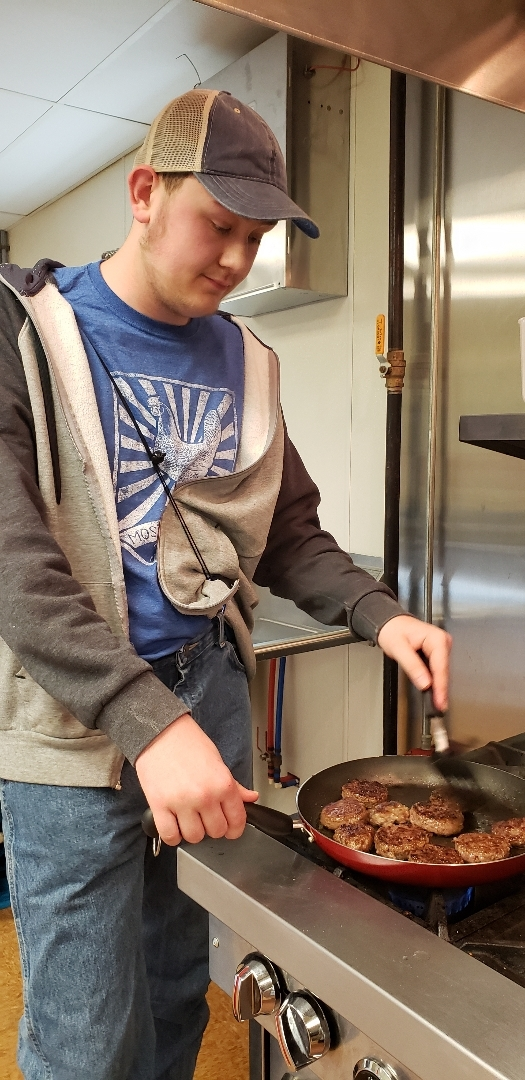## Welcome May!

This week has been nonstop jam making. We have made more jars of jam than we can count! We have also cooked lunch, worked on weeding, planted seeds, worked in the warehouse, started a tie dye project, and more. On a not so fun note, we have said our goodbyes to the Crestview and Mohawk classes. The school year is drawing to a close and so are the classroom visits. Only a few more days left with Leetonia and Salem. Then we will look forward to having all our friends return in the fall for harvest time! We will wrap up this week by attending the special needs production of Annie at Main Street Theater and having lunch out in town. Next week begins with our Whispering Pines visit and ends with a day at Camp Frederick. The month of May is exciting!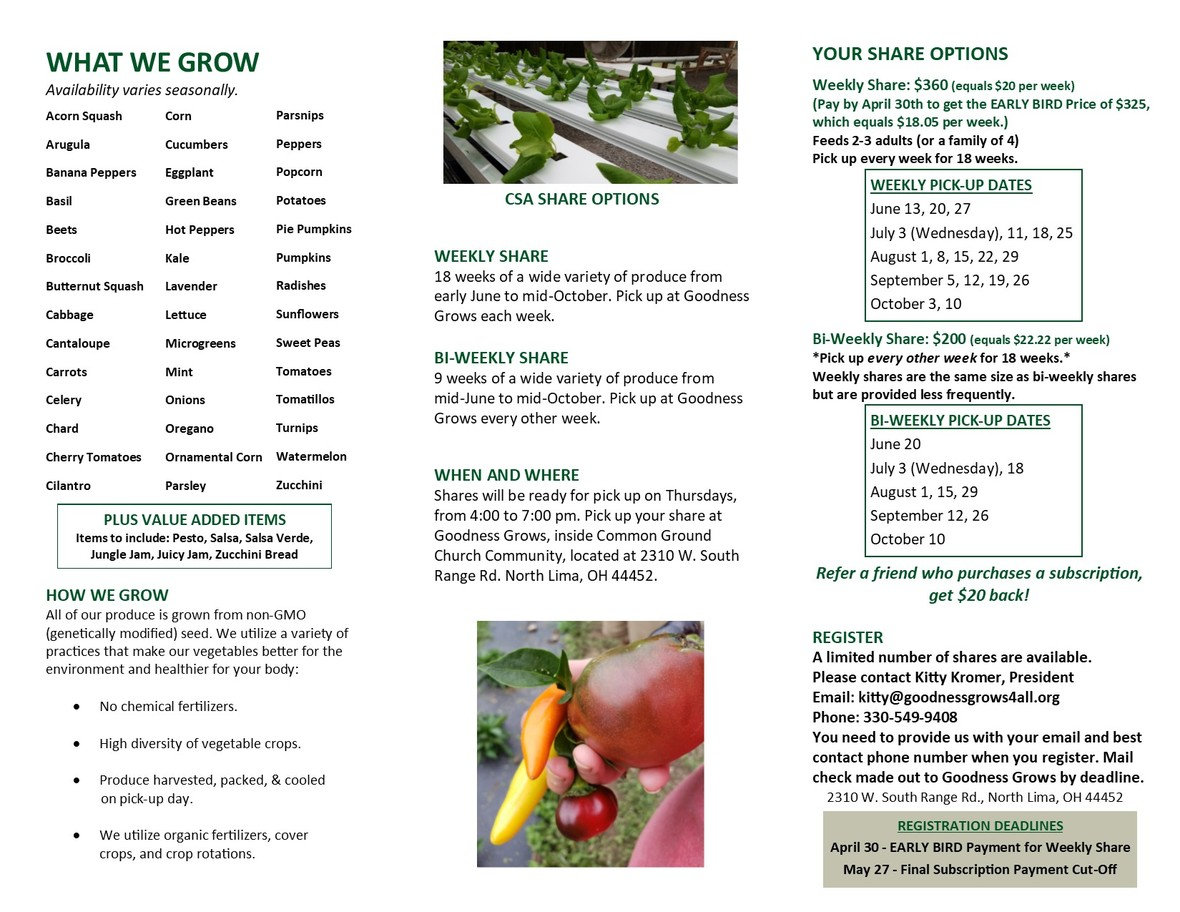## CSA Time!

A great big thank you to all our 2019 subscribers! We only have a few more spots left and the deadline is May 27th. Pick up is weekly, for 18 Thursdays, from early June to mid-October. The cost is \$360 for a weekly share, (\$20 per week). Or, do a bi-weekly share from mid-June to mid-October for \$200, (\$22.22 per week). Refer a friend who purchases a subscription, get \$20 back!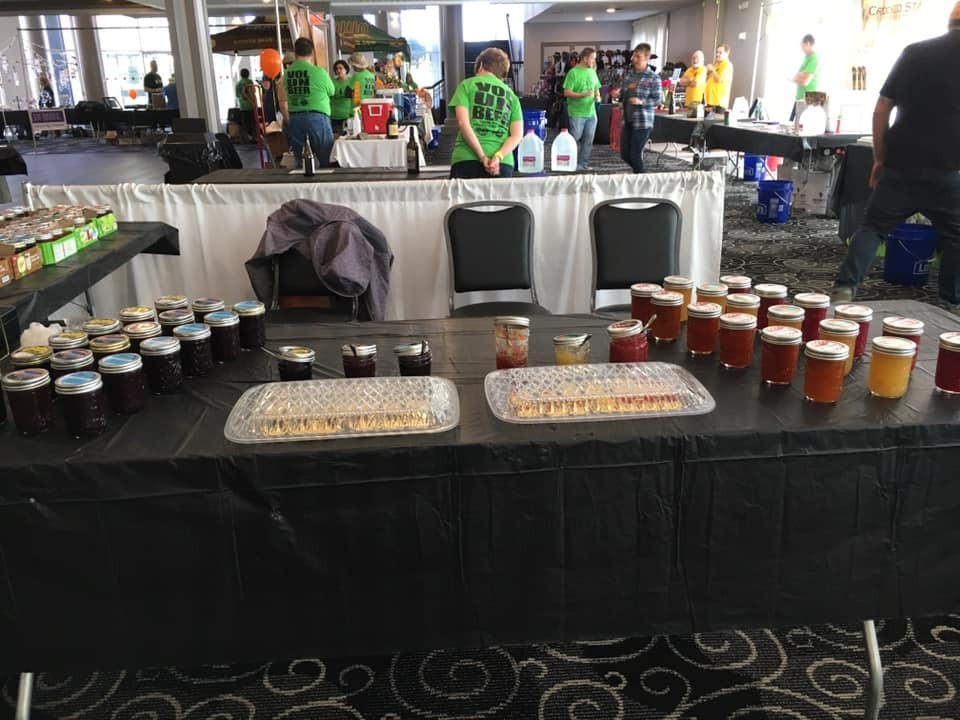## May Craft Shows & Markets

Spring weather means it's time for festivals and markets galore! Mark your calendars and come visit us out in the community for Jungle Jam, Juicy Jam, and (for a limited time) ferns.
May 3, 5-9pm ~ Austintown Farmers Market: Mother's Day Market
May 4, 10am-4pm ~ Heart & Home Marketplace, Summit Co. Fairgrounds
May 11, 9am-3pm ~ Youngstown Flea Mother's Day Market

# OUTREACH and MISSION OPPORTUNITIES

 table div table+table+table+table+table+table+table+table+table+table+table+table+table+table+table+table+table+table+table+table+table+table+table+table+table+table+table+table+table div table{width:100%;padding:0}table div table+table+table+table+table+table+table+table+table+table+table+table+table+table+table+table+table+table+table+table+table+table+table+table+table+table+table+table+table div table img{width:96.23%;padding:0;float:none}table div table+table+table+table+table+table+table+table+table+table+table+table+table+table+table+table+table+table+table+table+table+table+table+table+table+table+table+table+table div table td{width:100%;padding:0 1.88% 18px}/* styles */## Coat & Clothing Drive

Ongoing Outreach/Mission Opportunity
Drop items off in the collection barrel or take them up to the Green Room, anytime throughout the year! Let your friends and family know that we accept donations year-round. We are happy to take coats, clothes, shoes, purses and bags, jewelry, hats, gloves and mittens, scarves, and even small household items. Anything that is clean and in good repair that you think someone else might be able to use!
Date for Giveaway Set ~ Saturday, October 12th, 10am-1pm!

## Cards for Heroes

Last weekend to stop by the table in the Great Room to write a card for military personnel, veterans, and/or first responders! Help us spread some joy and appreciation. A simple card means more than you know! Grab a card, write a note, and then pop it into the appropriate envelope. More instructions are on the wall by the table. We will send off our missives of thanks in the beginning of May.

 /* styles */ ~~
 /* styles */ For I was hungry and you gave me food; I was thirsty and you gave me a drink; I was a stranger and you took me in; I was naked and you clothed me; I was sick and you visited me. I was in prison and you came to me. .....When you did it to one of the least of these my brothers and sisters, you were doing it to me. Matthew 25: 35-36, 40 ~ What can YOU do?
 table div table+table+table+table+table+table+table+table+table+table+table+table+table+table+table+table+table+table+table+table+table+table+table+table+table+table+table+table+table+table+table+table+table+table div table{width:100%;padding:0}table div table+table+table+table+table+table+table+table+table+table+table+table+table+table+table+table+table+table+table+table+table+table+table+table+table+table+table+table+table+table+table+table+table+table div table img{width:96.23%;padding:0;float:none}table div table+table+table+table+table+table+table+table+table+table+table+table+table+table+table+table+table+table+table+table+table+table+table+table+table+table+table+table+table+table+table+table+table+table div table td{width:100%;padding:0 1.88% 18px}/* styles */# LOCAL COMMUNITY NEWS/EVENTS

 /* styles */ If you know of any interesting news or events, please let us know, so that we can pass it on to others!

## Crown Theater Productions

Saturday and Sunday at 2pm
The big weekend is here! Don't miss out on this fun musical starring some of our favorite people, including Dustin Snyder as the Apple Seller and Tori Snyder as Usherette #1!

To the cast and crew we say "Break a leg!" Check out the picture below to see if you spot any familiar faces.

 table.module-37{width:0%;padding:0}table div table+table+table+table+table+table+table+table+table+table+table+table+table+table+table+table+table+table+table+table+table+table+table+table+table+table+table+table+table+table+table+table+table+table+table+table+table+table div table{width:0%;float:none;margin-left:auto;margin-right:auto;padding:0}table div table+table+table+table+table+table+table+table+table+table+table+table+table+table+table+table+table+table+table+table+table+table+table+table+table+table+table+table+table+table+table+table+table+table+table+table+table+table div table a{border:0 none;text-decoration:none}table div table+table+table+table+table+table+table+table+table+table+table+table+table+table+table+table+table+table+table+table+table+table+table+table+table+table+table+table+table+table+table+table+table+table+table+table+table+table div table img{width:100%!important;border:0 none;text-decoration:none}table div table+table+table+table+table+table+table+table+table+table+table+table+table+table+table+table+table+table+table+table+table+table+table+table+table+table+table+table+table+table+table+table+table+table+table+table+table+table div table td{width:100%;padding:0}/* styles */

## Canfield Presbyterian Curch Rummage Sale

 table.module-39{width:0%;padding:0}table div table+table+table+table+table+table+table+table+table+table+table+table+table+table+table+table+table+table+table+table+table+table+table+table+table+table+table+table+table+table+table+table+table+table+table+table+table+table+table+table div table{width:0%;float:none;margin-left:auto;margin-right:auto;padding:0}table div table+table+table+table+table+table+table+table+table+table+table+table+table+table+table+table+table+table+table+table+table+table+table+table+table+table+table+table+table+table+table+table+table+table+table+table+table+table+table+table div table a{border:0 none;text-decoration:none}table div table+table+table+table+table+table+table+table+table+table+table+table+table+table+table+table+table+table+table+table+table+table+table+table+table+table+table+table+table+table+table+table+table+table+table+table+table+table+table+table div table img{width:100%!important;border:0 none;text-decoration:none}table div table+table+table+table+table+table+table+table+table+table+table+table+table+table+table+table+table+table+table+table+table+table+table+table+table+table+table+table+table+table+table+table+table+table+table+table+table+table+table+table div table td{width:100%;padding:0}/* styles */

## Community Happenings at Mount Olivet

 table.module-41{width:0%;padding:0}table div table+table+table+table+table+table+table+table+table+table+table+table+table+table+table+table+table+table+table+table+table+table+table+table+table+table+table+table+table+table+table+table+table+table+table+table+table+table+table+table+table+table div table{width:0%;float:none;margin-left:auto;margin-right:auto;padding:0}table div table+table+table+table+table+table+table+table+table+table+table+table+table+table+table+table+table+table+table+table+table+table+table+table+table+table+table+table+table+table+table+table+table+table+table+table+table+table+table+table+table+table div table a{border:0 none;text-decoration:none}table div table+table+table+table+table+table+table+table+table+table+table+table+table+table+table+table+table+table+table+table+table+table+table+table+table+table+table+table+table+table+table+table+table+table+table+table+table+table+table+table+table+table div table img{width:100%!important;border:0 none;text-decoration:none}table div table+table+table+table+table+table+table+table+table+table+table+table+table+table+table+table+table+table+table+table+table+table+table+table+table+table+table+table+table+table+table+table+table+table+table+table+table+table+table+table+table+table div table td{width:100%;padding:0}/* styles */
 table div table+table+table+table+table+table+table+table+table+table+table+table+table+table+table+table+table+table+table+table+table+table+table+table+table+table+table+table+table+table+table+table+table+table+table+table+table+table+table+table+table+table+table div table{width:100%;padding:0}table div table+table+table+table+table+table+table+table+table+table+table+table+table+table+table+table+table+table+table+table+table+table+table+table+table+table+table+table+table+table+table+table+table+table+table+table+table+table+table+table+table+table+table div table img{width:96.23%;padding:0;float:none}table div table+table+table+table+table+table+table+table+table+table+table+table+table+table+table+table+table+table+table+table+table+table+table+table+table+table+table+table+table+table+table+table+table+table+table+table+table+table+table+table+table+table+table div table td{width:100%;padding:0 1.88% 18px}/* styles */# CELEBRATE!

May 5 - Cinco de Mayo
May 6 - National Nurses Day
May 7 - Pat & Jen Shannon
May 7 - National Teacher Day

 table.module-44{width:0%;padding:0}table div table+table+table+table+table+table+table+table+table+table+table+table+table+table+table+table+table+table+table+table+table+table+table+table+table+table+table+table+table+table+table+table+table+table+table+table+table+table+table+table+table+table+table+table+table div table{width:0%;float:none;margin-left:auto;margin-right:auto;padding:0}table div table+table+table+table+table+table+table+table+table+table+table+table+table+table+table+table+table+table+table+table+table+table+table+table+table+table+table+table+table+table+table+table+table+table+table+table+table+table+table+table+table+table+table+table+table div table a{border:0 none;text-decoration:none}table div table+table+table+table+table+table+table+table+table+table+table+table+table+table+table+table+table+table+table+table+table+table+table+table+table+table+table+table+table+table+table+table+table+table+table+table+table+table+table+table+table+table+table+table+table div table img{width:100%!important;border:0 none;text-decoration:none}table div table+table+table+table+table+table+table+table+table+table+table+table+table+table+table+table+table+table+table+table+table+table+table+table+table+table+table+table+table+table+table+table+table+table+table+table+table+table+table+table+table+table+table+table+table div table td{width:100%;padding:0}/* styles */

# ON THE CG CALENDAR

May 2-8. . .
Today, 10am - GG/Salem Class
Friday, 9am - GG at Annie
Friday, 5-9pm - GG at Austintown Market
Saturday, 10am-4pm - GG at Summit Co. Fairgrounds
Saturday & Sunday, 2pm - Annie at Crown Theater
Sunday, 11am - Worship
Sunday, 6-7:30pm - Youth Group
Monday, 9:30am - GG at Whispering Pines
Wednesday, 9:30am - Ladies Bible Study/* styles */ Seeking to reflect the unconditional love of God, our mission is to help people connect - to God, to one another, and to God's purpose for the world.
 table div table+table+table+table+table+table+table+table+table+table+table+table+table+table+table+table+table+table+table+table+table+table+table+table+table+table+table+table+table+table+table+table+table+table+table+table+table+table+table+table+table+table+table+table+table+table+table+table+table div table{width:100%;padding:0}table div table+table+table+table+table+table+table+table+table+table+table+table+table+table+table+table+table+table+table+table+table+table+table+table+table+table+table+table+table+table+table+table+table+table+table+table+table+table+table+table+table+table+table+table+table+table+table+table+table div table img{width:96.23%;padding:0;float:none}table div table+table+table+table+table+table+table+table+table+table+table+table+table+table+table+table+table+table+table+table+table+table+table+table+table+table+table+table+table+table+table+table+table+table+table+table+table+table+table+table+table+table+table+table+table+table+table+table+table div table td{width:100%;padding:0 1.88% 18px}/* styles *//* styles */ Come grow with us! Service Time: Sunday @ 11:00am Common Ground Church Community 2310 West South Range Road North Lima, Ohio 44452 ~~~~~~~~~~~~~~~~~~~~~~~~~~~~~~~~~~~~~~~~~~~~ For more information, call 330-549-9408 or contact us by email at commonground.secretary@gmail.com.
 /* styles */